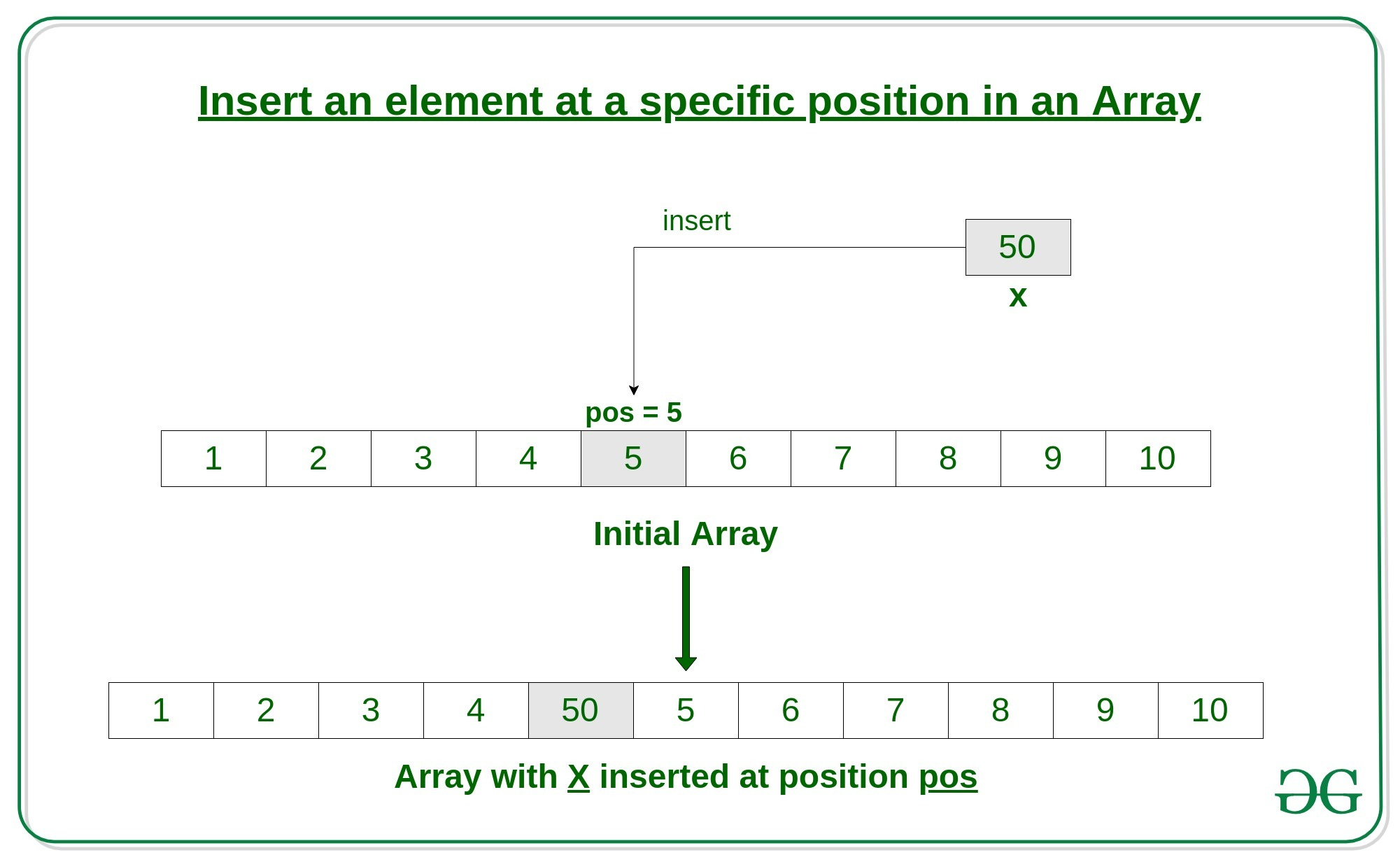# How to Insert an element at a specific position in an Array in C++

• Difficulty Level : Easy
• Last Updated : 21 Jun, 2022

An array is a collection of items stored at contiguous memory locations. In this article, we will see how to insert an element in an array in C++. Given an array arr of size n, this article tells how to insert an element x in this array arr at a specific position pos.Approach: Here’s how to do it.

1. First get the element to be inserted, say x
2. Then get the position at which this element is to be inserted, say pos
3. Then shift the array elements from this position to one position forward, and do this for all the other elements next to pos.
4. Insert the element x now at the position pos, as this is now empty.

Below is the implementation of the above approach:

## CPP

 `// C++ Program to Insert an element ` `// at a specific position in an Array `   `#include ` `using` `namespace` `std; `   `// Function to insert x in arr at position pos ` `int``* insertX(``int` `n, ``int` `arr[], ` `            ``int` `x, ``int` `pos) ` `{ ` `    ``int` `i; `   `    ``// increase the size by 1 ` `    ``n++; `   `    ``// shift elements forward ` `    ``for` `(i = n; i >= pos; i--) ` `        ``arr[i] = arr[i - 1]; `   `    ``// insert x at pos ` `    ``arr[pos - 1] = x; `   `    ``return` `arr; ` `} `   `// Driver Code ` `int` `main() ` `{ ` `    ``int` `arr = { 0 }; ` `    ``int` `i, x, pos, n = 10; `   `    ``// initial array of size 10 ` `    ``for` `(i = 0; i < 10; i++) ` `        ``arr[i] = i + 1; `   `    ``// print the original array ` `    ``for` `(i = 0; i < n; i++) ` `        ``cout << arr[i] << ``" "``; ` `    ``cout << endl; `   `    ``// element to be inserted ` `    ``x = 50; `   `    ``// position at which element is to be inserted ` `    ``pos = 5; `   `    ``// Insert x at pos ` `    ``insertX(n, arr, x, pos); `   `    ``// print the updated array ` `    ``for` `(i = 0; i < n + 1; i++) ` `        ``cout << arr[i] << ``" "``; ` `    ``cout << endl; `   `    ``return` `0; ` `} `

Output:

```1 2 3 4 5 6 7 8 9 10
1 2 3 4 50 5 6 7 8 9 10```

Time Complexity: O(n)

Auxiliary Space: O(1)

My Personal Notes arrow_drop_up
Recommended Articles
Page :